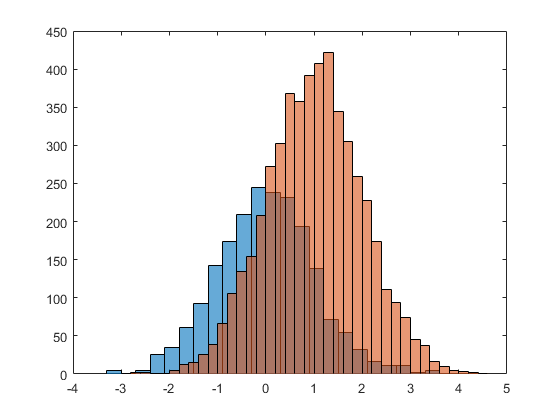# Multiple histograms from one TTree

Hello, in this root file WeTransfer - Send Large Files & Share Photos Online - Up to 2GB Free I stored in the TTree B1Scintparticles simulated data of particles hitting a scintillator. The branche ScintParticleKinEn is the kinetic energy of particles and the branch ScintParticleID is the Pdg code of the particle (for example ScintParticleID ==11 is an electron, ScintParticleID==-11 is a positron and ScintParticleID ==22 is a photon).

By this macro

I can plot the kinetic energy of a specif particle, for example:
scintillator.cpp (3.2 KB)

``````TCut Idpele = TString::Format("ScintParticleID==11").Data();
t->Draw(hstring,Idpele);
``````

I plot the kinetic energy of electrons.

Now, i would plot the kinetic energy of each particle in the same plot …i.e. something like thisSo, I define the 3 TCut (one for each particles kind)

``````TCut Idpele = TString::Format("ScintParticleID==11").Data();
TCut Idppos = TString::Format("ScintParticleID==-11").Data();
TCut Idpgam = TString::Format("ScintParticleID==22").Data();
``````

and I’ve to plot the

1. kinetic energy using the TCut Idpele
2. kinetic energy using the TCut Idppos
3.kinetic energy using the TCut Idgam

I tried writing a macro but it crashes…

scintillator.cpp (3.2 KB)

can someone help me to fix it please?

ROOT Version: Not Provided
Platform: Not Provided
Compiler: Not Provided

If you are filling the histograms with tree->Draw, you can save each Draw to a different histogram, e.g. 3 lines like this (of course, using h1, h2, h3 and cut1, cut2, cut3, resp.):

``````tree->Draw("something >> h1...","cut 1");
``````

and then put the 3 histos in a THStack.

scintillator.cpp (3.6 KB)

``````void scintillator() {
float fillcolor=0.35;
gStyle->SetOptStat(111110);
TFile *fin = TFile::Open("B1.root");

TTree *t=0;
fin->GetObject("B1Scintparticles",t);
t->SetScanField(0);
TCanvas *c01 = new TCanvas("c01","",1280,1024);

auto h1 = new TH1D("h1","h1",100., 0., 1.); h1->SetFillColor(kRed);
auto h2 = new TH1D("h2","h2",100., 0., 1.); h2->SetFillColor(kRed+1);
auto h3 = new TH1D("h3","h3",100., 0., 1.); h3->SetFillColor(kRed+2);

TString helestring = TString::Format("ScintParticleKinEn >> h1");
TString hposstring = TString::Format("ScintParticleKinEn >> h2");
TString hgamstring = TString::Format("ScintParticleKinEn >> h3");

TCut Idpele = TString::Format("ScintParticleID==11").Data();
TCut Idppos = TString::Format("ScintParticleID==-11").Data();
TCut Idpgam = TString::Format("ScintParticleID==22").Data();

t->Draw(helestring,Idpele,"goff");
t->Draw(hposstring,Idppos,"goff");
t->Draw(hgamstring,Idpgam,"goff");

THStack *hs = new THStack();
hs->Draw();
}
``````

Hello @couet thank you, your code works and I get this graph (it looks like different from your graph because here on root forum I just uploaded a semple of few simulated events…)

But I’ve still 2 problems:

1. I need the x-axis and y-axis title, so I added the code
``````hs->GetXaxis()->SetTitle("Energy (GeV)");
hs->GetYaxis()->SetTitle("Counts");
hs->GetXaxis()->SetLabelFont(43);
hs->GetXaxis()->SetLabelSize(30);
hs->GetYaxis()->SetLabelFont(43);
hs->GetYaxis()->SetLabelSize(30);
hs->GetXaxis()->SetTitleFont(43);
hs->GetXaxis()->SetTitleSize(30);
hs->GetYaxis()->SetTitleFont(43);
hs->GetYaxis()->SetTitleSize(30);
``````
``````but the macro crashes. I also tried
``````
``````h1->GetXaxis()->SetTitle("Energy (GeV)");
h1->GetYaxis()->SetTitle("Counts");
h1->GetXaxis()->SetLabelFont(43);
h1->GetXaxis()->SetLabelSize(30);
h1->GetYaxis()->SetLabelFont(43);
h1->GetYaxis()->SetLabelSize(30);
h1->GetXaxis()->SetTitleFont(43);
h1->GetXaxis()->SetTitleSize(30);
h1->GetYaxis()->SetTitleFont(43);
h1->GetYaxis()->SetTitleSize(30);
``````

in this case the macro doesn’t crash, but I don’t get the axes titles…

1. I need to know how many electrons, positrons and photons hit the scintillator then I wanted to print the TPavestats to read the entries, but even if I don’t have` SetStats(0)` in the macro, if write the code
``````TPaveStats *stats = (TPaveStats*)h1->GetListOfFunctions()->FindObject("stats");
TPaveStats *stats2 = (TPaveStats*)h2->GetListOfFunctions()->FindObject("stats");
TPaveStats *stats3 = (TPaveStats*)h3->GetListOfFunctions()->FindObject("stats");
stats->SetTextColor(kBlue);
stats->SetX1NDC(0.80); stats->SetX2NDC(0.98);
stats->SetY1NDC(0.77); stats->SetY2NDC(0.92);
stats2->SetTextColor(kGreen);
stats2->SetX1NDC(0.80); stats->SetX2NDC(0.98);
stats2->SetY1NDC(0.60); stats->SetY2NDC(0.75);
stats3->SetTextColor(kYellow);
stats3->SetX1NDC(0.80); stats->SetX2NDC(0.98);
stats3->SetY1NDC(0.43); stats->SetY2NDC(0.58);
``````

The macro crashes…
scintillator.cpp (3.7 KB)

``````void scintillator() {
float fillcolor=0.35;
gStyle->SetOptStat(111110);
TFile *fin = TFile::Open("B1.root");

TTree *t=0;
fin->GetObject("B1Scintparticles",t);
t->SetScanField(0);
TCanvas *c01 = new TCanvas("c01","",1280,1024);

auto h1 = new TH1D("h1","h1",100., 0., 1.); h1->SetFillColor(kRed);
auto h2 = new TH1D("h2","h2",100., 0., 1.); h2->SetFillColor(kRed+1);
auto h3 = new TH1D("h3","h3",100., 0., 1.); h3->SetFillColor(kRed+2);

TString helestring = TString::Format("ScintParticleKinEn >> h1");
TString hposstring = TString::Format("ScintParticleKinEn >> h2");
TString hgamstring = TString::Format("ScintParticleKinEn >> h3");

TCut Idpele = TString::Format("ScintParticleID==11").Data();
TCut Idppos = TString::Format("ScintParticleID==-11").Data();
TCut Idpgam = TString::Format("ScintParticleID==22").Data();

t->Draw(helestring,Idpele,"goff");
t->Draw(hposstring,Idppos,"goff");
t->Draw(hgamstring,Idpgam,"goff");

THStack *hs = new THStack();
hs->Draw();

auto *pt = new TPaveText(.3,.8,.5,.89,"NDC NB");
pt->AddText(Form("Number of entries in h1 : %f",h1->GetEntries()));
pt->AddText(Form("Number of entries in h2 : %f",h2->GetEntries()));
pt->AddText(Form("Number of entries in h3 : %f",h3->GetEntries()));
pt->Draw();

hs->GetXaxis()->SetTitle("Energy (GeV)");
hs->GetYaxis()->SetTitle("Counts");
hs->GetXaxis()->SetLabelFont(43);
hs->GetXaxis()->SetLabelSize(30);
hs->GetYaxis()->SetLabelFont(43);
hs->GetYaxis()->SetLabelSize(30);
hs->GetXaxis()->SetTitleFont(43);
hs->GetXaxis()->SetTitleSize(30);
hs->GetYaxis()->SetTitleFont(43);
hs->GetYaxis()->SetTitleSize(30);
}
``````

1 Like
``````{
TH1F *h1 = new TH1F("h1", "h1 for a test hstack", 10, -4, 4);
h1->FillRandom("gaus", 20000); h1->SetFillColor(kRed);
TH1F *h2 = new TH1F("h2", "h2 for a test hstack", 10, -4, 4);
h2->FillRandom("gaus", 15000); h2->SetFillColor(kBlue);
TH1F *h3 = new TH1F("h3", "h3 for a test hstack", 10, -4, 4);
h3->FillRandom("gaus", 10000); h3->SetFillColor(kGreen);
TCanvas *c = new TCanvas("c", "c");
// first, draw all histograms so that their "stats" boxes are created
TPaveStats *s = 0;
s = (TPaveStats*)h1->FindObject("stats");
if (s) { s->SetY1NDC(s->GetY1NDC() - 0.1); s->SetY2NDC(s->GetY2NDC() - 0.1); }
s = (TPaveStats*)h2->FindObject("stats");
if (s) { s->SetY1NDC(s->GetY1NDC() - 0.3); s->SetY2NDC(s->GetY2NDC() - 0.3); }
s = (TPaveStats*)h3->FindObject("stats");
if (s) { s->SetY1NDC(s->GetY1NDC() - 0.5); s->SetY2NDC(s->GetY2NDC() - 0.5); }
// then, create and draw the hstack
THStack *hs = new THStack("hs", ""); // note: an empty title